# Problem: What is the value of the rate constant k for this reaction?For the reaction A + B + C → D + E, the initial reaction rate was measured for various initial concentrations of reactants. The following data were collected:Trial()()()Initial Rate()10.100.100.1020.100.100.3030.200.100.1040.200.200.10

###### FREE Expert Solution

order with respect to [A]

x = 1 → first order

order with respect to [B]

y = 0 → zeroth order

order with respect to [C]

z = 1 → first order

83% (404 ratings)###### Problem Details

What is the value of the rate constant k for this reaction?

For the reaction A + B + C → D + E, the initial reaction rate was measured for various initial concentrations of reactants. The following data were collected:

 Trial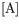(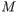)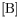()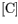() Initial Rate(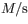) 1 0.10 0.10 0.10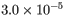2 0.10 0.10 0.30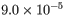3 0.20 0.10 0.10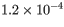4 0.20 0.20 0.10